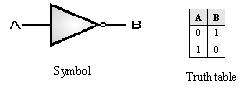Home > VHDL > Logic Gates > NOT Gate

Figure below shows the symbol and the truth table of the NOT gate. The NOT gate can be implemented using the data flow modelling or the behavioural modelling. In data flow modelling of NOT gate the operator NOT is used to logically invert the input A. In behavioural modelling the behaviour of the gate is explained with the help of sequential statements.library IEEE;

use IEEE.std_logic_1164.all;

entity NOT2 is

port (A : in std_logic;

B : out std_logic);

end NOT2;

architecture data_flow of NOT2 is

begin

B <= NOT A;

end data_flow;

library IEEE;

use IEEE.std_logic_1164.all;

entity NOT2 is

port (A : in std_logic;

B : out std_logic);

end NOT2;

architecture behav of NOT2 is

begin

process (A)

begin

if A='0' then

B <= '1';

else B <= '0';

end if;

end process;

end behav;